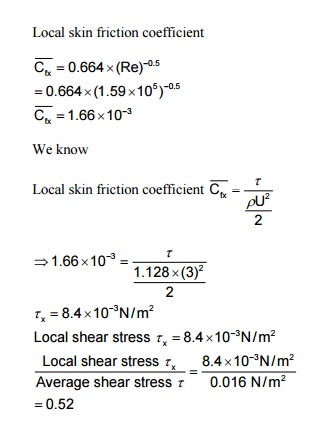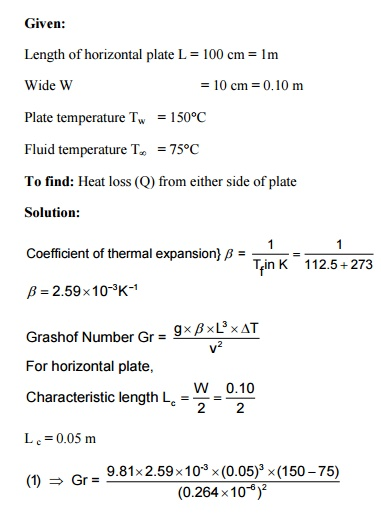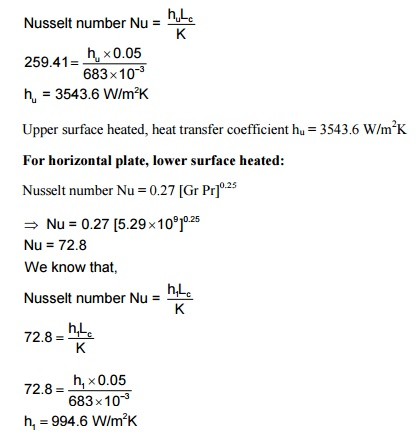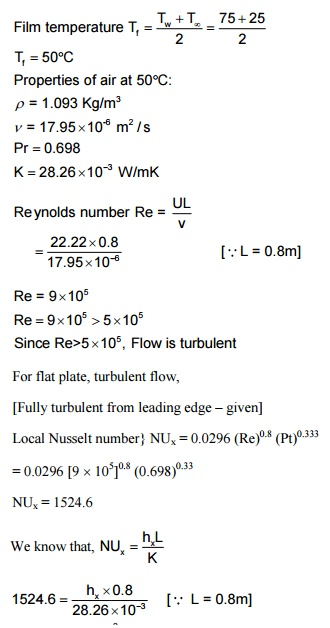Home | | Heat and Mass Transfer | Solved Problems - Heat and Mass Transfer - Convection

# Solved Problems - Heat and Mass Transfer - Convection

Mechanical - Heat and Mass Transfer - Convection

1. Air at 20C at atmospheric pressure flows over a flat plate at a velocity of 3 m/s. if the plate is 1 m wide and 80C, calculate the following at x = 300 mm.

1.       Hydrodynamic boundary layer thickness,

2.       Thermal boundary layer thickness,

3.       Local friction coefficient,

4.       Average friction coefficient,

5. Local heat transfer coefficient

6. Average heat transfer coefficient,

7. Heat transfer.

Given: Fluid temperature T¥ = 20°C , Velocity U = 3 m/s

Wide W = 1 m

Surface temperature Tw = 80°C

Distance x = 300 mm = 0.3 m

Solution: We know6. Average heat transfer coefficient (h):

h =2 ´hx =2 ´6.20

h =12.41 W / m2K

7. Heat transfer:

We know that,

Q =h A(Tw   -T¥)

= 12.41´(1 ´0.3) (80-20) Q = 23.38 Watts

2.       Air at 40°C is flows over a flat plate of 0.9 m at a velocity of 3 m/s. Calculate the following:

1.     Overall drag coefficient

2.     Average shear stress,

3.     Compare the average shear stress with local shear stress (shear stress at the trailing edge)

Given:

Fluid temperature T¥ = 40°C

Length L = 0.9 m

Velocity U = 3 m/s.

Solution:

Properties of air at 40°C:

r=1.128 Kg/m3

n= 16.96 ´10-6 m2 / s Pr =0.699

= 26.56  ´10-3  W/mK3.       Air at 20oC flows over a flat plate at 60oC with a free stream velocity of 6 m/s. Determine the value of the average convective heat transfer coefficient up to a length of 1 m in the flow direction.

in the flow direction.    (AU2013)

Given:

Fluid temperature Tinf = 20o

Plate temperature Tw = 60o

Velocity   U = 6 m/s

Length  L = 1 m

To find: Average heat transfer coefficient

Solution: We knowLocal nusselt number} NUx =4.65 W/m2K Average heat transfer coefficient} h = 2 ´hx =2 ´4.65

h =9.31 W/m2K

4. Engine oil flows through a 50 mm diameter tube at an average temperature of 147°C.

The flow velocity is 80 cm/s. Calculate the average heat transfer coefficient if the tube wall is maintained at a temperature of 200°C and it is 2 m long.

Given:

Diameter D    = 50 mm = 0.050 m

Average temperature Tm= 147°C

Velocity U= 80 cm/s = 0.80 m/s

Tube wall temperature Tw= 200°C

Length L= 2m

To find: Average heat transfer coefficient (h)

Solution: Properties of engine oil at 147°C

r= 816 Kg/m3 n= 7 ´10-6 m2 / s Pr = 116

K  = 133.8 ´10-3  W/mK

We know5. A thin 100 cm long and 10 cm wide horizontal plate is maintained at a uniform temperature of 150°C in a large tank full of water at 75°C. Estimate the rate of heat to be supplied to the plate to maintain constant plate temperature as heat is dissipated from either side of plate.Gr = 3.41´109

Gr Pr = 3.41´109   ´1.55

Gr Pr = 5.29 ´109

Gr Pr value is in between 8 ´106 and 1011

i.e., 8 ´106< G r Pr < 1011

For horizontal plate, upper surface heated:

Nusselt number Nu = 0.15 (Gr Pr)0.333

Þ   Nu = 0.15 [5.29 ´109 ]0.333+

Þ   Nu = 259.41

We know that,Lower surface heated, heat transfer coefficient h1 = 994.6 W/m2K

Total heat transfer Q = (hu + h1) ´A ´DT

= (hu + h1) ´W ´L ´(Tw - T¥)

= (3543.6 + 994.6) ´0.10 ´(150 –75) Q = 34036.5 W

6. For a particular engine, the underside of the crank case can be idealized as a flat plat measuring 80 cm ´20 cm. The engine runs at 80 km/hr and the crank case is cooled by air flowing past it at the same speed. Calculate the loss of heat from the crank case surface of temperature 75°C to the ambient air temperature 25°C. Assume the boundary layer becomes turbulent from the loading edge itself.

Given: Area A = 80 cm ´20 cm

= 1600 cm2 = 0.16m2

Velocity U = 80 Km/hr

=80 ´10 3  / m 3600s

=22.22 m/sSurface temperature Tw =75 C° Ambient air temperature T¥ =25 C°

Flow is turbulent from the leading edge, flow is fully turbulent.

To find:hx  =53.85 W/m2K

Local heat transfer coefficient} hx = 53.85 W/m2K

For turbulent flow, flat plate

Average heat transfer coefficient} h = 1.24 hx h = 1.24 ´53.85

h = 66.78 W/m2K We know,

Heat loss Q = h A (Tw - T¥) = 66.78 ´0.16 (75 –25)

Q = 534.2 watts.

Study Material, Lecturing Notes, Assignment, Reference, Wiki description explanation, brief detail
Mechanical : Heat and Mass Transfer : Convection : Solved Problems - Heat and Mass Transfer - Convection |